# ML Aggarwal Solutions Class 10 Maths Chapter 17 Mensuration

Exercise 17.1

Take π = 22/7 unless stated otherwise.
1. Find the total surface area of a solid cylinder of radius 5 cm and height 10 cm. Leave your answer in terms of π.
Solution:
Given radius of the cylinder, r = 5 cm
Height of the cylinder, h = 10 cm
Now, as we know that
Total surface area = 2r(r+h)
= 2×5(5+10)
= 2×5×15
= 150 cm2
Hence, the total surface area of the solid cylinder is 150 cm2.

2. An electric geyser is cylindrical in shape, having a diameter of 35 cm and height 1.2m. Neglecting the thickness of its walls, calculate
(i) its outer lateral surface area,
(ii) its capacity in litres.
Solution:
We have given that:
Given diameter of the cylinder, d = 35 cm
radius, r = d/2 = 35/2 = 17.5 cm
Height of the cylinder, h = 1.2 m = 120 cm
Now,
(i) Outer lateral surface area = 2rh
= 2×(22/7)×17.5×120
= 13200 cm2
Therefore, the outer lateral surface area =13200 cm2
(ii) as we know that,
Capacity of the cylinder = r2 h
= (22/7)×17.52×120
= 115500 cm3
= 115.5 litres [∵1000 cm3  = 1 litre]
Therefore, the capacity of the cylinder =115.5 litres.
Hence the outer lateral surface area and the capacity of the cylinder is 13200 cm2 and 115.5 litres respectively.

3. A school provides milk to the students daily in cylindrical glasses of diameter 7 cm. If the glass is filled with milk upto a height of 12 cm, find how many litres of milk is needed to serve 1600 students.
Solution:
As we have given that:
Diameter of the cylindrical glass, d = 7 cm
Radius, r = d/2 = 7/2 = 3.5 cm
Height of the cylindrical glass, h = 12 cm
Volume of the cylindrical glass, V = r2 h
= (22/7)×3.52×12
= 462 cm3
∴ Number of students = 1600
To find the Milk needed for 1600 students
= 1600×462
= 739200 cm3
= 739.2 litres [∵1000 cm3  = 1 litre]
Hence the amount of milk needed to serve 1600 students is 739.2 litres.

4. In the given figure, a rectangular tin foil of size 22 cm by 16 cm is wrapped around to form a cylinder of height 16 cm. Find the volume of the cylinder.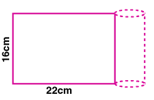Solution:
We have given that:
Height of the cylinder, h = 16 cm
When rectangular foil of length 22 cm is folded to form cylinder, the base circumference of the cylinder is 22cm
2r = 22
r = 22/2
= 3.5 cm
Now, Volume of the cylinder, V = r2 h
= (22/7)×3.52×16
= 616 cm3
Hence the volume of the cylinder is 616 cm3.

5. (i) How many cubic metres of soil must be dug out to make a well 20 metres deep and 2 metres in diameter?
(ii) If the inner curved surface of the well in part (i) above is to be plastered at the rate of Rs 50 per m2, find the cost of plastering.
Solution:
(i) Given diameter of the well, d = 2 m
Radius, r = d/2 = 2/2 = 1 m
Depth of the well, h = 20 m
Volume of the well, V = r2 h
= (22/7)×12×20
= 62.85 m3
Hence the amount of soil dug out to make the well is 62.85 m3.
(ii)Curved surface area of the well = 2rh
= 2×(22/7)×1×20
= 880/7 m2
Cost of plastering the well per m = Rs.50
Total cost of plastering the well = 50×(880/7)
= Rs.6285.71
Hence the total cost of plastering is Rs.6285.71.

6. A road roller (in the shape of a cylinder) has a diameter 0.7 m and its width is 1.2 m. Find the least number of revolutions that the roller must make in order to level a playground of size 120 m by 44 m.
Solution:
We have given that:
Given diameter of the road roller, d = 0.7 m
Radius, r = d/2 = 0.7/2 = 0.35 m
Width, h = 1.2 m
Curved surface area of the road roller = 2rh
=2 ×(22/7)×0.35×1.2
= 2.64 m2
Area of the play ground = l×b
= 120×44
= 5280 m2
Number of revolutions = Area of play ground / Curved surface area
= 5280/2.64
= 2000
Hence the road roller must take 2000 revolutions to level the ground.

7. If the volume of a cylinder of height 7 cm is 448 π cm3, find its lateral surface area and total surface area.
Solution:
We have given that:
Given Height of the cylinder, h = 7 cm
Volume of the cylinder, V = 448 cm3
r2 h = 448
×r2×7 = 448
r2  = 448/7 = 64
r = 8
Lateral surface area = 2rh
= 2××8×7
= 112 cm2
Total surface area = 2r(r+h)
= 2××8×(8+7)
= 2××8×15
= 240 cm2
Hence the lateral surface area and the total surface area of the cylinder are 112 cm2 and 240 cm2.

8. A wooden pole is 7 m high and 20 cm in diameter. Find its weight if the wood weighs 225 kg per m3.
Solution:
We have given that:
Given height of the pole, h = 7 m
Diameter of the pole, d = 20 cm
radius, r = d/2 = 20/2 = 10 cm = 0.1m
Volume of the pole = r2 h
= (22/7)×0.12×7
= 0.22 m3
Weight of wood per m3  = 225 kg
weight of 0.22 m3 wood = 225×0.22 = 49.5 kg
Hence the weight of the wood is 49.5 kg.

9. The area of the curved surface of a cylinder is 4400 cm2, and the circumference of its base is 110 cm. Find
(i) the height of the cylinder.
(ii) the volume of the cylinder.
Solution:
We have given that:
Given curved surface area of a cylinder = 4400 cm2
Circumference of its base = 110 cm
2r = 110
r = 110/2 = (110×7)/2×22 = 17.5 cm
(i) Curved surface area of a cylinder, 2rh = 4400
2×(22/7)×17.5×h = 4400
h = (4400×7)/2×22×17.5
= 40 cm
Hence the height of the cylinder is 40 cm.
(ii)Volume of the cylinder, V = r2 h
= (22/7)×17.52×40
= 38500 cm3
Hence the volume of the cylinder is 38500 cm3.

10. A cylinder has a diameter of 20 cm. The area of curved surface is 1000 cm2. Find
(i) the height of the cylinder correct to one decimal place.
(ii) the volume of the cylinder correct to one decimal place. (Take π = 3.14)
Solution:
We have given that:
(i) Given diameter of the cylinder, d = 20 cm
Radius, r = d/2 = 20/2 = 10 cm
Curved surface area = 1000 cm2
2rh = 1000
2×3.14×10×h = 1000
62.8h = 1000
h = 1000/62.8
= 15.9 cm
Hence the height of the cylinder is 15.9 cm.
(ii)Volume of the cylinder, V = r2 h
= 3.14×102×15.9
= 4992.6 cm3
Hence the volume of the cylinder is 4992.6  cm3

11. The barrel of a fountain pen, cylindrical in shape, is 7 cm long and 5 mm in diameter. A full barrel of ink in the pen will be used up when writing 310 words on an average. How many words would use up a bottle of ink containing one-fifth of a litre?
Answer correct to the nearest. 100 words.
Solution:
We have given that:
Height of the barrel of a pen, h = 7 cm
Diameter, d = 5mm = 0.5 cm
Radius, r = d/2 = 0.5/2 = 0.25 cm
Volume of the barrel of pen, V = r2 h
= (22/7)×0.252×7
= 1.375 cm3
Ink in the bottle, = one fifth of a litre
= (1/5)×1000 = 200 ml
Number of words written using full barrel of ink = 310
Number of words written by using this ink = (200/1.375)×310 = 45090.90 words
Round off to nearest hundred, we get 45100 words.
Hence the number of words written using the ink is 45100 words.

12. Find the ratio between the total surface area of a cylinder to its curved surface area given that its height and radius are 7.5 cm and 3.5 cm.
Solution:
We have given that:
Given radius of the cylinder, r = 3.5 cm
Height of the cylinder, h = 7.5 cm
Total surface area = 2r(r+h)
Curved surface area = 2rh
Ratio of Total surface area to curved surface area = 2r(r+h)/ 2rh
= (r+h)/h
= (3.5+7.5)/7.5
= 11/7.5
= 22/15
Hence the required ratio is 22:15.

13. The radius of the base of a right circular cylinder is halved and the height is doubled. What is the ratio of the volume of the new cylinder to that of the original cylinder?
Solution:
We have given that:
Let the radius of the base of a right circular cylinder be r and height be h.
Volume of the cylinder, V1  = r2 h
The radius of the base of a right circular cylinder is halved and the height is doubled.
So radius of new cylinder = r/2
Height of new cylinder = 2h
Volume of the new cylinder, V2  = r2 h
= (r/2)2  ×2h
= ½ r2 h
So ratio of volume of new cylinder to the original cylinder , V2/V1= ½ r2 h/r2 h = ½
Hence the required ratio is 1:2.

14. (i) The sum of the radius and the height of a cylinder is 37 cm and the total surface area of the cylinder is 1628 cm2 . Find the height and the volume of the cylinder.
(ii) The total surface area of a cylinder is 352 cm2 . If its height is 10 cm, then find the diameter of the base.
Solution:
We have given that:
(i) Let r be the radius and h be the height of the cylinder.
Given the sum of radius and height of the cylinder,r+h = 37 cm
Total surface area of the cylinder = 1628 cm2
2r(r+h) = 1628
2×(22/7)×r×37 = 1628
r = (1628×7)/(2×22×37)
r = 7 cm
We have r+h = 37
7+h = 37
h = 37-7 = 30 cm
Volume of the cylinder, V = r2 h
= (22/7)×72×30
= (22/7)×49×30
= 4620 cm3
Hence the height and volume of the cylinder is 30 cm and 4620 cm3 respectively.
(ii) Total surface area of the cylinder = 353 cm2
Height, h = 10 cm
2r(r+h) = 352
2×(22/7)×r×(r+10) = 352
r2+10r = (352×7)/2×22
r2+10r = 56
r2+10r-56 = 0
(r+14)(r-4) = 0
r+14 = 0 or r-4 = 0
r = -14 or r = 4
Radius cannot be negative. So r = 4.
Diameter = 2×r = 2×4 = 8 cm.
Hence the diameter of the base is 8 cm.

15.The ratio between the curved surface and the total surface of a cylinder is 1∶ 2. Find the volume of the cylinder, given that its total surface area is 616 cm2.
Solution:
We have given that:
Given the ratio of curved surface area and the total surface area = 1:2
Total surface area = 616 cm2
Curved surface area = 616/2 = 308 cm2
2rh = 308
rh = 308/2
= 308×7/2×22
rh = 49 …(i)
Total surface area, 2rh+2r2  = 616
308+2r2  = 616
2r2  = 616-308
2r2  = 308
r2  = 308/2 = 154
r2  = 154/ = 154×7/22 = 49
Taking square root on both sides
r = 7
Substitute r in (i)
rh = 49
7×h = 49
h = 49/7 = 7 cm
Volume of the cylinder, V = r2h
= (22/7)×72×7
= 1078 cm3
Hence the volume of the cylinder is 1078 cm3.

16. Two cylindrical jars contain the same amount of milk. If their diameters are in the ratio 3∶ 4, find the ratio of their heights.
Solution:
We have given that:
Let r1 and r2 be the radius of the two cylinders and h1 and h2 be their heights.
Given ratio of the diameter = 3:4
Then the ratio of radius r1:r2  = 3:4
Given volume of both jars are same.
r12h1 = r22h2
h1/h2 = r22/ r12
h1/h2 = 42/32  = 16/9
Hence the ratio of the heights are 16:9.

17. A rectangular sheet of tin foil of size 30 cm × 18 cm can be rolled to form a cylinder in two ways along length and along breadth. Find the ratio of volumes of the two cylinders thus formed.
Solution:
We have given that:
Given size of the sheet = 30 cm×18 cm
If we roll it lengthwise, base circumference, 2r = 30
2×(22/7)r = 30
r = 30×7/2×22 = 210/44 = 105/22 cm
Height, h = 18 cm
Volume of the cylinder, V1  = r2 h
= (22/7)×(105/22)2×18
= 15×105×9/11
If we roll it breadthwise, base circumference, 2r = 18
2×(22/7)r = 18
r = 18×7/2×22 = 126/44 = 63/22 cm
Height, h = 30 cm
Volume of the cylinder, V2  = r2 h
= (22/7)×(63/22)2×30
= 9×63×15/11
V1/V2  = (15×105×9/11)÷( 9×63×15/11)
= (15×105×9/11)×(11/9×63×15)
= 105/63
= 15/9
= 5/3
Ratio of the volumes of two cylinders is 5:3.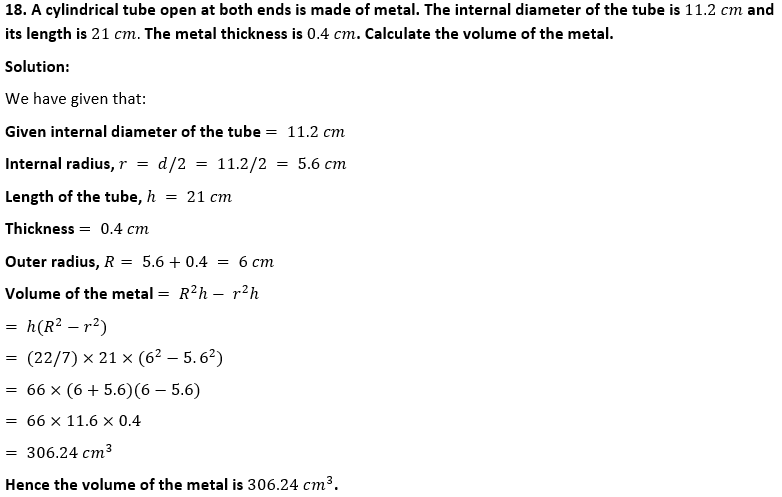19. The given figure shows a metal pipe 77 cm long. The inner diameter of a cross-section is 4 cm and the outer one is 4.4 cm. Find its
(i) inner curved surface area
(ii) outer curved surface area
(iii) total surface area.Solution:
We have given that:
Given height of the metal pipe = 77 cm
Inner diameter = 4 cm
Inner radius, r = d/2 = 4/2 = 2 cm
Outer diameter = 4.4 cm
Outer radius, R = d/2 = 4.4/2 = 2.2 cm
(i)Inner curved surface area = 2rh
= 2×(22/7)×2×77
= 968 cm2
Hence the inner surface area is 968 cm2.
(ii)Outer curved surface area = 2Rh
= 2×(22/7)×2.2×77
= 1064.8 cm2
Hence the outer curved surface area is 968 cm2.
(iii)Area of ring = (R2-r2)
= (22/7)×(2.22-22)
= (22/7)×(4.84-4)
= (22/7)×0.84
= 2.64
Total surface area = inner surface area + outer surface area + area of two rings
= 968+ 1064.8+ 2×2.64
= 2038.08 cm2
Hence the total surface area of the metal pipe is 2038.08 cm2.

20. A lead pencil consists of a cylinder of wood with a solid cylinder of graphite filled in the interior. The diameter of the pencil is 7 mm and the diameter of the graphite is 1 mm. If the length of the pencil is 14 cm, find the volume of the wood and that of the graphite.
Solution:
We have given that:
Given length of the pencil, h = 14 cm
Diameter of the pencil = 7 mm
radius, R = 7/2 mm = 7/20 cm
Diameter of the graphite = 1 mm
Radius of graphite, r = ½ mm = 1/20cm
Volume of graphite = r2 h
= (22/7)×(1/20)2×14
= 11/100
= 0.11 cm3
Hence the volume of the graphite is 0.11 cm3
Volume of the wood = (R2-r2)h
= (22/7)×(7/20)2-(1/20)2]14
= (22/7)×[(49/400)-(1/400)]14
= (22/7)×(48/400)×14
= 11×12/25
= 5.28 cm3
Hence the volume of the wood is 5.28 cm3

21. A soft drink is available in two packs
(i) a tin can with a rectangular base of length 5 cm and width 4 cm, having a height of 15 cm and
(ii) a plastic cylinder with circular base of diameter 7 cm and height 10 cm.
Which container has greater capacity and by how much?
Solution:
We have given that:
(i) Length of the can, l = 5 cm
Width, b = 4 cm
Height, h = 15 cm
Volume of the can = lbh
= 5×4×15
= 300 cm3
(ii) Diameter of the cylinder, d = 7 cm
Radius, r = d/2 = 7/2 = 3.5 cm
Height, h = 10 cm
Volume of the cylinder, V = r2 h
= (22/7)×3.52×10
= 385 cm3
Difference of the volume = 385-300 = 85 cm3
Hence the plastic cylinder has 85 cm3 more volume than the tin can.

22. A cylindrical roller made of iron is 2 m long. Its inner diameter is 35 cm and the thickness is 7 cm all round. Find the weight of the roller in kg, if 1 cm3 of iron weighs 8 g.
Solution:
We have given that:
Length of the roller, h = 2 m = 200 cm [1m = 100 cm]
Inner diameter = 35 cm
Inner radius, r = 35/2 cm
Thickness = 7 cm
= (35+14)/2
= 49/2 cm
Volume of the iron in roller = (R2-r2)h
= (22/7)[( 49/2)2-(35/2)2]200
= (22/7)[( 492-352/4]200
= (22/7)×50(492-352)
= (22/7)×50( 492-352)
= (22/7)×50 (2401-1225)
= (22/7)×50×1176
= 184800 cm^3
Given 1 cm^3 of iron weighs 8 g.
Weight of the roller = 184800×8 = 1478400 g
= 1478.4 kg [1 kg = 1000 g]
Hence the weight of the roller is 1478.4 kg.

Exercise 17.2

Take π = 22/7 unless stated otherwise.

1. Write whether the following statements are true or false. Justify your answer.
(i) If the radius of a right circular cone is halved and its height is doubled, the volume will remain unchanged.
(ii) A cylinder and a right circular cone are having the same base radius and same height. The volume of the cylinder is three times the volume of the cone.
(iii) In a right circular cone, height, radius and slant height are always the sides of a right triangle.
Solution:
(i)Volume of cone = (1/3)r2 h
If radius is halved and height is doubled, then volume = (1/3)(r/2)2  2h = (1/3)r2 h/2
If the radius of a right circular cone is halved and its height is doubled, the volume will be halved.
So given statement is false.
Height of cylinder = height of cone
Volume of cylinder = r2 h
Volume of cone = (1/3)r2 h
The volume of the cylinder is three times the volume of the cone.

So given statement is true.
(iii)In a right circular cone,
l2= h2+r2
In a right circular cone, height, radius and slant height are always the sides of a right triangle.
So given statement is true.

2. Find the curved surface area of a right circular cone whose slant height is 10 cm and base radius is 7 cm.
Solution:
We have given that:
Given slant height of the cone, l = 10 cm
Base radius, r = 7 cm
Curved surface area of the cone = rl
= (22/7)×7×10 = 220 cm2
Hence the curved surface area of the cone is 220 cm2 .

3. Diameter of the base of a cone is 10.5 cm and slant height is 10 cm. Find its curved surface area.
Solution:
We have given that:
Given diameter of the cone = 10.5 cm
Radius, r = d/2 = 10.5/2 = 5.25 cm
Slant height of the cone, l = 10 cm
Curved surface area of the cone = rl
= (22/7)×5.25×10 = 165 cm2
Hence the curved surface area of the cone is 165 cm2 .

4. Curved surface area of a cone is 308 cm2  and its slant height is 14 cm. Find ,
(ii)total surface area of the cone.
Solution:
(i) Given curved surface area of the cone = 308 cm2
Slant height of the cone, l = 14 cm
rl = 308
(22/7)×r×14 = 308
r = 308×7/(22×14)
= 7
Hence the radius of the cone is 7 cm.
(ii)Total surface area of the cone = Base area + curved surface area
= r2+ rl
= (22/7)×72+308
= (22/7)×49 +308
= 154+308
= 462
Hence the total surface area of the cone is 462 cm2.

5. Find the volume of the right circular cone with
(i) radius 6 cm and height 7 cm
(ii) radius 3.5 cm and height 12 cm.
Solution:
We have given that:
(i)Given radius, r = 6 cm
Height, h = 7 cm
Volume of the cone = (1/3)r2 h
=(1/3)×(22/7)×62×7
= 22×12
= 264 cm3
Hence the volume of the cone is 264 cm3.
(ii) Given radius, r = 3.5 cm
Height, h = 12 cm
Volume of the cone = (1/3)r2 h
=(1/3)×(22/7)×3.52×12
= (22/7)×12.25×4
= 154 cm3
Hence the volume of the cone is 154 cm3.

6. Find the capacity in litres of a conical vessel with
(i) radius 7 cm, slant height 25 cm
(ii) height 12 cm, slant height 13 cm
Solution:
We have given that:
Given radius, r = 7 cm
Slant height, l = 25 cm
We know that l2  = h2+r2Volume of the cone = (1/3)r2 h
=(1/3)×(22/7)×72×24
= 22×7×8
= 1232 cm3
= 1.232 litres [1 litre = 1000 cm3]
Hence the volume of the cone is 1.232 litres.
(ii) Given height, h = 12 cm
Slant height, l = 13 cm
We know that l2  = h2+r2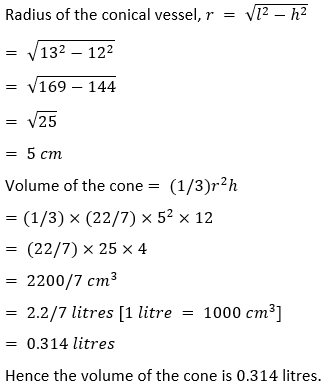7. A conical pit of top diameter 3.5 m is 12 m deep. What is its capacity in kiloliters ?
Solution:
We have given that:
Given diameter, d = 3.5 m
So radius, r = 3.5/2 = 1.75
Depth, h = 12 m
Volume of the cone = (1/3)r2 h
=(1/3)×(22/7)×1.752×12
= (22/7)× 1.752×4
= 38.5 m3
= 38.5 kilolitres [1 kilolitre = 1m3]
Hence the volume of the conical pit is 38.5 kilolitres.

8. If the volume of a right circular cone of height 9 cm is 48π cm3 , find the diameter of its base.
Solution:
We have given that:
Given height of a cone, h = 9 cm
Volume of the cone = 48
(1/3)r2 h = 48
(1/3)r2×9 = 48
3r2  = 48
r2  = 48/3 = 16
r = 4
= 2×4
= 8 cm
Hence the diameter of the cone is 8 cm.

9. The height of a cone is 15 cm. If its volume is 1570 cm2 , find the radius of the base. (Use π = 3.14)
Solution:
Given height of a cone, h = 15 cm
Volume of the cone = 1570 cm3
(1/3)r2 h = 1570
(1/3)3.14 ×r2×15 = 1570
5 ×3.14×r2  = 1570
r2  = 1570/5×3.14 = 314/3.14 = 100
r = 10
Hence the radius of the cone is 10 cm.

10. The slant height and base diameter of a conical tomb are 25 m and 14 m respectively. Find the cost of white washing its curved surface area at the rate of Rs 210 per 100 m2  .
Solution:
Given slant height of conical tomb, l = 25 m
Base diameter, d = 14 m
So radius, r = 14/2 = 7 m
Curved surface area = rl
= (22/7)×7×25 = 550 m2
Hence the curved surface area of the cone is 550 m2 .
Rate of washing its curved surface area per 100 m2   = Rs.210
So total cost = (550/100)×210 = Rs.1155
Hence the total cost of washing its curved surface area is Rs.1155.

11. A conical tent is 10 m high and the radius of its base is 24 m. Find :
(i) slant height of the tent.
(ii) cost of the canvas required to make the tent, if the cost of 1 m2  canvas is Rs 70.
Solution:
We have given that:
(i)Given height of the tent,h = 10 m
We know that l2  = h2+r2
l2= 102+242
l2= 100+576
l2= 676
l = √676
l = 2612. A Jocker’s cap is in the form of a right circular cone of base radius 7 cm and height 24 cm. Find the area of the cloth required to make 10 such caps.
Solution:
We have given that:
Given height of the cone, h = 24 cm
We know that l2  = h2+r2
l2  = 242+72
l2  = 576+49
l2  = 625
l = √625
l = 25
Curved surface area = rl
= (22/7)×7×25
= 22×25
= 550 cm2
So the area of the cloth required to make 10 such caps = 10×550 = 5500
Hence the area of the cloth required to make 10 caps is 5500 cm2.

13. (a) The ratio of the base radii of two right circular cones of the same height is 3∶ 4. Find the ratio of their volumes.
(b) The ratio of the heights of two right circular cones is 5∶ 2 and that of their base radii is 2∶ 5. Find the ratio of their volumes.
(c) The height and the radius of the base of a right circular cone is half the corresponding height and radius of another bigger cone. Find:
(i) the ratio of their volumes.
(ii) the ratio of their lateral surface areas.
Solution:
We have given that:
(a) Let r1 and r2 be the radius of the given cones and h be their height.Hence the ratio of the volumes is 2:5.
(c) Let r be the radius of bigger cone. Then the radius of smaller cone is r/2.
Let h be the height of bigger cone. Then the height of smaller cone is h/2.
(i)Volume of bigger cone, V1  = (1/3)r2 h
Volume of smaller cone, V = (1/3)(r/2)2  (h/2) = (1/3)r2 h/8
Ratio of volume of smaller cone to bigger cone, V2/V1  = ( 1/3)r2  h/8÷ (1/3)r2 h
= (1/24)r2 h ×(3/r2  h)
= 1/8
Hence the ratio of their volumes is 1:8.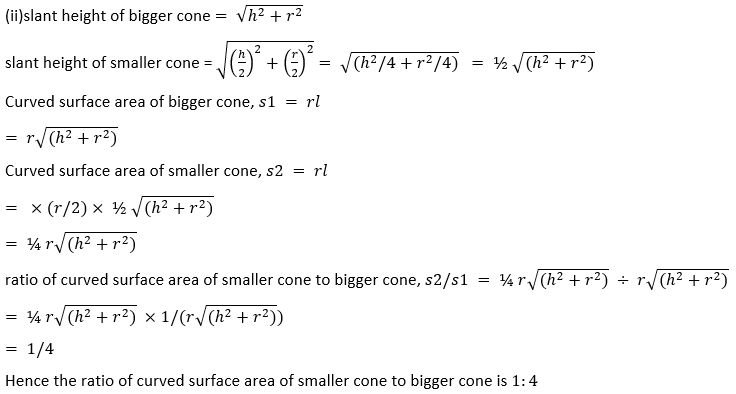14. Find what length of canvas 2 m in width is required to make a conical tent 20 m in diameter and 42 m in slant height allowing 10% for folds and the stitching. Also find the cost of the canvas at the rate of Rs 80 per metre.
Solution:
We have given that:
Given diameter of the conical tent, d = 20 m
radius, r = d/2 = 20/2 = 10 m
Slant height, l = 42 m
Curved surface area of the conical tent = rl
= (22/7)×10×42
= 22×10×6
= 1320 m2
So the area of canvas required is 1320 m2.
Since 10% of this area is used for folds and stitches, actual cloth needed = 1320+ 10% of 1320
= 1320 + (10/100)×1320
= 1320+132
= 1452 m2
Width of the cloth = 2m
Length of the cloth = Area/width = 1452/2 = 726 m
Cost of canvas = Rs.80 per metre.
Total cost = 80×726 = Rs.58080
Hence the total cost of the canvas is Rs.58080.

15. The perimeter of the base of a cone is 44 cm and the slant height is 25 cm. Find the volume and the curved surface of the cone.
Solution:
We have given that:
Given perimeter of the base of a cone = 44 cm
2r = 44
2×22/7×r = 44
r = 44×7/(2×22)
r = 7 cm
Slant height, l = 25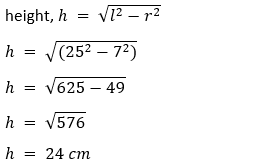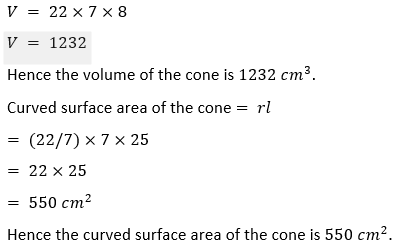16. The volume of a right circular cone is 9856 cm3  and the area of its base is 616 cm2 . Find
(i) the slant height of the cone.
(ii) total surface area of the cone.
Solution:
Given base area of the cone = 616 cm2
r2  = 616
(22/7)×r2  = 616
r2  = 616×7/22
r2  = 196
r = 14
Given volume of the cone = 9856 cm3
(1/3)r2 h = 9856
(1/3)×(22/7)×142  ×h = 9856
h = (9856×3×7)/(22×142)
h = (9856×3×7)/(22×196)
h = 4817. A right triangle with sides 6 cm,8 cm and 10 cm is revolved about the side 8 cm. Find the volume and the curved surface of the cone so formed. (Take π = 3.14)
Solution:
We have given that:So the height of the resulting cone, h = 8 cm
Slant height, l = 10 cm
Volume of the cone, V = (1/3)r2 h
V = (1/3)×3.14×6^2×8
V = (1/3)×3.14×36×8
V = 3.14×12×8
V = 301.44 cm3
Hence the volume of the cone is 301.44 cm3.
Curved surface area of the cone = rl
= 3.14×6×10
= 188.4
Hence the curved surface area of the cone is 188.4 cm2.

18. The height of a cone is 30 cm. A small cone is cut off at the top by a plane parallel to its base. If its volume be 1/27 of the volume of the given cone, at what height above the base is the section cut?
Solution:
We have given that:
Given height of the cone, H = 30 cm
Let R be the radius of the given cone and r be radius of small cone.
Let h be the height of small cone.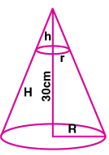Volume of the given cone = (1/3)R2 H
Volume of the small cone = 1/27 th of the volume of the given cone.
(1/3)r2 h = (1/27)× (1/3)R2 H
Substitute H = 30
(1/3)r2 h = (1/27)× (1/3)R2×30
r2 h/R2  = 30/27
r2 h/R2= 10/9 ….(i)
From figure, r/R = h/H
r/R = h/30 ….(ii)
Substitute (ii) in (i)
(h/30)2×h = 10/9
h3/900 = 10/9
h3= 900×10/9 = 1000
Taking cube root on both sides.
h = 10 cm
H-h = 30-10 = 20
The small cone is cut at a height of 20 cm above the base.

19. A semi-circular lamina of radius 35 cm is folded so that the two bounding radii are joined together to form a cone. Find
(i) the radius of the cone.
(ii) the (lateral) surface area of the cone.
Solution:
We have given that: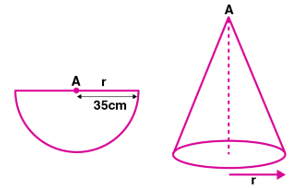(i)Given radius of the semi circular lamina, r = 35 cm
A cone is formed by folding it.
So the slant height of the cone, l = 35 cm
Let r1 be radius of cone.
Semicircular perimeter of lamina becomes the base of the cone.
r = 2 r1
r = 2  r1
35 = 2  r1
r1   = 35/2= 17.5 cm
Hence the radius of the cone is 17.5 cm.
(ii) Curved surface area of the cone =  r1  l
= (22/7)×17.5×35
= 22×17.5×5
= 1925 cm2
Hence the lateral surface area of the cone is 1925 cm2.

Exercise 17.3

1. Find the surface area of a sphere of radius :
(i) 14 cm
(ii) 10.5 cm
Solution:
(i) Given radius of the sphere, r = 14 cm
Surface area of the sphere = 4r2
= 4×(22/7)×142
= 4×22×14×2
= 2464 cm3
Hence the surface area of the sphere is 2464 cm2.
(ii) Given radius of the sphere, r = 10.5 cm
Surface area of the sphere = 4r2
= 4×(22/7)×10.52
= 1386 cm3
Hence the surface area of the sphere is 1386 cm2.

2. Find the volume of a sphere of radius :
(i) 0.63 m
(ii) 11.2 cm
Solution:
(i) Given radius of the sphere, r = 0.63 m
Volume of the sphere, V = (4/3)r3
= (4/3)×(22/7)×0.633
= 1.047 m3
= 1.05 m3 (approx)
Hence the volume of the sphere is 1.05 m3.
(ii) Given radius of the sphere, r = 11.2 cm
Volume of the sphere, V = (4/3)r3
= (4/3)×(22/7)×11.23
= 5887.317 cm3
= 5887.32 cm3 (approx)
Hence the volume of the sphere is 5887.32 cm3.

3. Find the surface area of a sphere of diameter:
(i) 21 cm
(ii) 3.5 cm
Solution:
We have given that:
(i) Given diameter of the sphere, d = 21 cm
Radius, r = d/2 = 21/2 = 10.5
Surface area of the sphere = 4r2
= 4×(22/7)×10.52
= 1386 cm2
Hence the surface area of the sphere is 1386 cm2.
(ii) Given diameter of the sphere, d = 3.5 cm
Radius, r = d/2 = 3.5/2 = 1.75
Surface area of the sphere = 4r2
= 4×(22/7)×1.752
= 38.5 cm2
Hence the surface area of the sphere is 38.5 cm2.

4. A shot-put is a metallic sphere of radius 4.9 cm. If the density of the metal is 7.8 g per cm3, find the mass of the shot-put.
Solution:
We have given that:
Given radius of the metallic sphere,r = 4.9 cm
Volume of the sphere, V = (4/3)r3
V = (4/3)×(22/7)×4.9
V = 493.005
V = 493 cm^3  (approx)
Given Density = 7.8 g per cm3
Density = Mass/ Volume
Mass = Density × Volume
= 7.8×493
= 3845.4 g
Hence the mass of the shot put is 3845.4 g.

5. Find the diameter of a sphere whose surface area is 154 cm2 .
Solution:
We have given that:
Given surface area of the sphere = 154 cm2
Surface area of the sphere = 4r2
4×(22/7)×r2  = 154
r2  = 154 ×7/(22×4)
= 49/4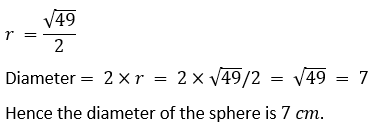6. Find:
(i) the curved surface area.
(ii) the total surface area of a hemisphere of radius 21 cm.
Solution:
(i) Given radius of the hemisphere, r = 21 cm
Curved surface area of the hemisphere = 2r2
= 2×(22/7)×212
= 2×22×3×21
= 2772 cm2
Therefore, the curved surface area of the hemisphere is 2772 cm2.
(ii)Total surface area of the hemisphere = 3r2
= 3×(22/7)×212
= 3×22×3×21
= 4158 cm2
Hence the total surface area of the hemisphere is 4158 cm2.

7. A hemispherical brass bowl has inner- diameter 10.5 cm. Find the cost of tin-plating it on the inside at the rate of Rs 16 per 100 cm2.
Solution:
We have given that:
Given inner diameter of the brass bowl, d = 10.5 cm
Radius, r = d/2 = 10.5/2 = 5.25 cm
Curved surface area of the bowl = 2r2
= 2×(22/7)×5.252
= 173.25 cm2
Rate of tin plating = Rs.16 per 100 cm2
So total cost = 173.25×16/100 = 27.72
Hence the cost of tin plating the bowl on the inside is Rs.27.72.

8. The radius of a spherical balloon increases from 7 cm to 14 cm as air is jumped into it. Find the ratio of the surface areas of the balloon in two cases.
Solution:
We have given that:
Given radius of the spherical balloon, r = 7 cm
Radius of the spherical balloon after air is pumped, R = 14 cm
Surface area of the sphere = 4r2
Ratio of surface areas of the balloons = 4r2/4R2
= r2/R2
= 72/142
= 1/4
Hence the ratio of the surface areas of the spheres is 1:4.

9. A sphere and a cube have the same surface area. Show that the ratio of the volume of the sphere to that of the cube is √6 ∶√π
Solution:
We have given that:
Let r be the radius of the sphere and a be the side of the cube.
Surface area of sphere = 4r2
Surface area of cube = 6a2
Given sphere and cube has same surface area.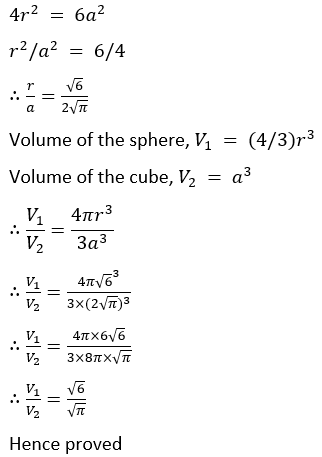10. (a) If the ratio of the radii of two sphere is 3∶ 7, find :
(i) the ratio of their volumes.
(ii) the ratio of their surface areas.
(b) If the ratio of the volumes of the two sphere is 125∶ 64, find the ratio of their surface areas.
Solution:
(i)Let the radii of two spheres be r1 and r2.
Given ratio of their radii = 3:7
Volume of sphere = (4/3)r3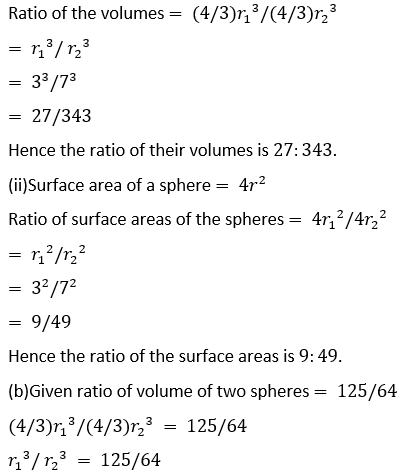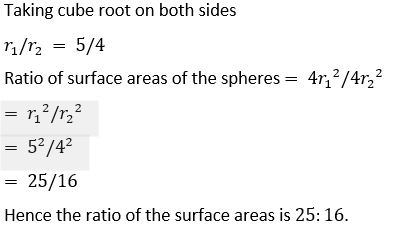11. A cube of side 4 cm contains a sphere touching its sides. Find the volume of the gap in between.
Solution:
Given side of the cube, a = 4 cm
Volume of the cube = a3
= 4^3
= 4×4×4
= 64 cm3
Diameter of the sphere = 4 cm
So radius of the sphere, r = d/2 = 4/2 = 2 cm
Volume of the sphere = (4/3)r3
= (4/3)×(22/7)×23
= 33.523
= 33.52 cm3 (approx)
Volume of the gap in between = 64 – 33.52
= 30.48
= 30.5 cm3 (approx)
Hence the volume of the gap between the cube and sphere is 30.5 cm3.

12. Find the volume of a sphere whose surface area is 154 cm2.
Solution:
We have given that:
Given surface area of the sphere = 154 cm2
4r2  = 154
4×(22/7)×r2  = 154
r2  = (154×7)/(4×22)
r2  = 49/4
r = 7/2
Volume of the sphere = (4/3)r3
= (4/3)×(22/7)×(7/2)3
= 539/3
= 179.666
= 179.67 cm3 (approx)
Hence the volume of the sphere is 179.67 cm3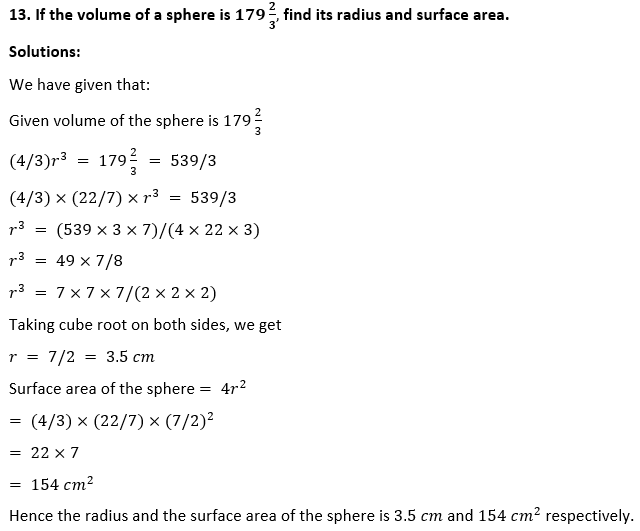14. A hemispherical bowl has a radius of 3.5 cm. What would be the volume of water it would contain?
Solution:
We have given that:
Given radius of the hemispherical bowl, r = 3.5 cm = 7/2 cm
Volume of the hemisphere = (2/3)r3
= (2/3)×(22/7)×(7/2)3
= 11×49/6
= 539/6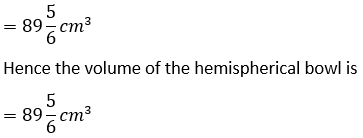15. The water for a factory is stored in a hemispherical tank whose internal diameter is 14 m. The tank contains 50 kilolitres of water. Water is pumped into the tank to fill to its capacity. Find the volume of water pumped into the tank.
Solution:
We have given that:
Given internal diameter of the hemispherical tank, d = 14 m
So radius, r = 14/2 = 7 m
Volume of the tank = (2/3)r3
= (2/3)×(22/7)×(7)3
= 718.667 m3
= 718.67 m3 (approx)
= 718.67 kilolitre
Quantity of water in tank = 50 kilolitre
Amount of water to be pumped into the tank = 718.67-50 = 668.67 kilolitre.
Hence the volume of water pumped into the tank is 668.67 kilolitre.

16. The surface area of a solid sphere is 1256 cm². It is cut into two hemispheres. Find the total surface area and the volume of a hemisphere. Take π = 3.14.
Solution:
We have given that:
Given surface area of the sphere = 1256 cm2
4r2   = 1256
4×3.14×r2  = 1256
r2   = 1256×/3.14×4
r2 = 100
r = 10 cm
Total surface area of the hemisphere = 3r2
= 3×3.14×102
= 3×3.14×100
= 942 cm2
Hence the total surface area of the hemisphere is 942 cm2.
Volume of the hemisphere = (2/3)r3
= (2/3)×3.14×103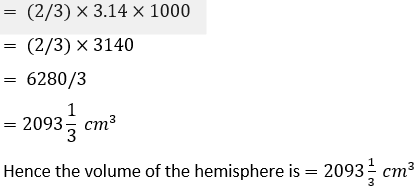17. Write whether the following statements are true or false. Justify your answer :
(i) The volume of a sphere is equal to two-third of the volume of a cylinder whose height and diameter are equal to the diameter of the sphere.
(ii) The volume of the largest right circular cone that can be fitted in a cube whose edge is 2r equals the volume of a hemisphere of radius r.
(iii) A cone, a hemisphere and a cylinder stand on equal bases and have the same height. The ratio of their volumes is 1∶ 2∶ 3.
Solution:
We have given that:
(i)Let the radius of sphere be r.
Then height of the cylinder, h = 2r
Volume of cylinder = r2 h
= ×r2×2r
= 2r3
Volume of sphere = (4/3)r3
= (2/3)× 2r3
= (2/3)× Volume of cylinder
Hence the given statement is true.
(ii)Let the edge of the cube is 2r.
So radius of cone = r
Height of cone, h = 2r
Volume of cone = (1/3)r2 h
= (1/3)r2×2r
= (2/3)r3
= Volume of a hemisphere of radius r
Hence the given statement is true.
(iii)Let r be radius of cone, hemisphere and cylinder.
So the height of the cone = r
Height of cylinder = r
Volume of cone = (1/3)r2 h
= (1/3)r3
Volume of hemisphere = (2/3)r3
Volume of cylinder = r2 h
= r
Ratio of volume of cone , hemisphere and cylinder = (1/3)r3  : (2/3)r3  : r3
= 1/3∶ 2/3∶ 1
= 1:2:3
Hence the given statement is true.

Exercise 17.4

Take π = 22/7 unless stated otherwise.
1. The adjoining figure shows a cuboidal block of wood through which a circular cylindrical hole of the biggest size is drilled. Find the volume of the wood left in the block.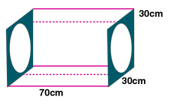Solution:
We have given that:
Given diameter of the hole, d = 30 cm
radius of the hole, r = d/2 = 30/2 = 15 cm
Height of the cylindrical hole, h = 70 cm
Volume of the cuboidal block = lbh
= 70×30×30
= 63000 cm3
Volume of cylindrical hole = r2 h
= (22/7)×152×70
= 22×225×10
= 49500 cm3
Volume of the wood left in the block = 63000-49500 = 13500 cm3
Hence the volume of the wood left in the block is 13500 cm3.

2. The given figure shows a solid trophy made of shining glass. If one cubic centimetre of glass costs Rs 0.75, find the cost of the glass for making the trophy.Solution:
We have given that:
Given side of the cube, a = 28 cm
Radius of the cylinder, r = 28/2 = 14 cm
Height of the cylinder, h = 28 cm
Volume of the cube = a3
= 283
= 28×28×28
= 21952 cm3
Volume of the cylinder = r2 h
= (22/7)×142×28
= 22×2×14×28
= 17248 cm3
Total volume of the solid = Volume of the cube + volume of the cylinder
= 21952+17248
= 39200 cm3
Cost of 1 cm3 glass = Rs.0.75
Total cost of glass = 39200×0.75
= Rs.29400
Hence the cost of making the trophy is Rs.29400.

3. From a cube of edge 14 cm, a cone of maximum size is carved out. Find the volume of the remaining material.
Solution:
We have given that: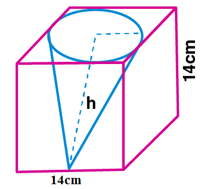Given edge of the cube, a = 14 cm
Radius of the cone, r = 14/2 = 7 cm
Height of the cone, h = 14 cm
Volume of the cube = a3
= 143
= 14×14×14
= 2744 cm3
Volume of the cone = (1/3)r2 h
= (1/3)×(22/7)×72×14
= 22×7×14/3
= 2156/3 cm3
Volume of the remaining material = Volume of the cube- Volume of the cone
= 2744-2156/3
= (3×2744-2156)/3
= (8232 – 2156)/3
= 6076/3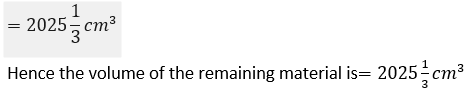4. A cone of maximum volume is curved out of a block of wood of size 20 cm x 10 cm x 10 cm. Find the volume of the remaining wood.
Solution:
We have given that: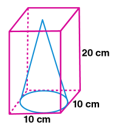Given dimensions of the block of wood = 20 cm × 10 cm× 10 cm
Volume of the block of wood = 20×10×10 = 2000 cm3 [Volume = lbh]
Diameter of the cone, d = 10 cm
Radius of the cone , r = d/2 = 10/2 = 5 cm
Height of the cone, h = 20 cm
Volume of the cone = (1/3)r2 h
= (1/3)×(22/7)×52×20
= (22×25×20)/(3×7)
= 11000/21 cm3
Volume of the remaining wood = Volume of block of wood – Volume of cone
= 2000-11000/21
= (21×2000-11000)/21
= (42000-11000)/21
= 31000/21
= 1476.19 cm3
Hence the volume of the remaining wood is 1476.19 cm3.

5. 16 glass spheres each of radius 2 cm are packed in a cuboidal box of internal dimensions 16 cm × 8 cm × 8 cm and then the box is filled with water. Find the volume of the water filled in the box.
Solution:
We have given that:
Given dimensions of the box = 16 cm ×8 cm ×8 cm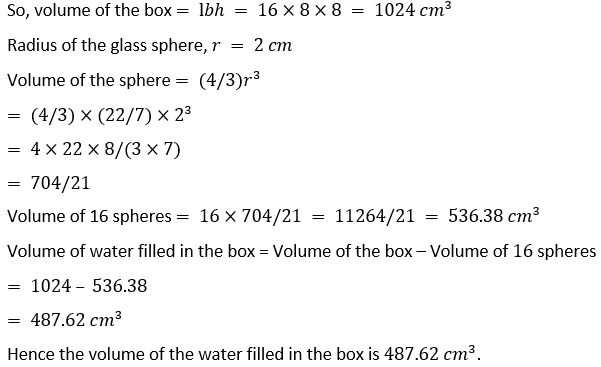6. A pen stand made of wood is in the shape of a cuboid with four conical depressions to hold pens. The dimensions of the cuboid are 15 cm by 10 cm by 3.5 cm. The radius of each of the depression is 0.5 cm and the depth is 1.4 cm. Find the volume of the wood in the entire stand, correct to 2 decimal places.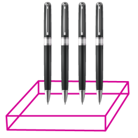Solution:
Dimensions of the cuboid = 15 cm× 10 cm × 3.5 cm
Volume of the cuboid = 15×10×3.5 = 525 cm3
Radius of each depression, r = 0.5 cm
Depth, h = 1.4 cm
Volume of conical depression = (1/3)r2 h
= (1/3)×(22/7)×0.52×1.4
= 22×0.25×1.4/21
= 7.7/21 cm3
Volume of 4 such conical depressions = 4×7.7/21
= 1.467 cm3
Volume of wood in the stand = Volume of the cuboid- Volume of 4 conical depressions
= 525-1.467
= 523.533
= 523.53 cm3
Hence the volume of the wood in the stand is 523.53 cm3.

7. A cubical block of side 7 cm is surmounted by a hemisphere. What is the greatest diameter that the hemisphere can have? Also, find the surface area of the solid.
Solution:
We have given that:Given edge of the cube, a = 7 cm
Diameter of the hemisphere, d = 7 cm
Radius, r = d/2 = 7/2 = 3.5 cm
Surface area of the hemisphere = 2r2
= 2×(22/7)×3.52
= 44×12.25/7
= 539/7
= 77 cm2
Surface area of the cube = 6a2
= 6×72
= 6×49
= 294 cm2
Surface area of base of hemisphere = r2
= (22/7)×3.52
= 22×12.25/7
= 38.5 cm2
Surface area of the solid = surface area of the cube + surface area of hemisphere – surface area of the base of hemisphere
= 294+77-38.5
= 332.5 cm2
Hence the surface area of the solid is 332.5 cm2.

8. A wooden article was made by scooping out a hemisphere from each end of a solid cylinder (as shown in the given figure). If the height of the cylinder is 10 cm and its base is of radius 3.5 cm, find the total surface area of the article.Solution:
We have given that:
Given height of the cylinder, h = 10 cm
Radius of the cylinder, r = 3.5 cm
Radius of the hemisphere = 3.5 cm
Total surface area of the article = curved surface area of the cylinder + curved surface area of 2 hemispheres
= 2rh + 2× 2r2
= 2r(h+2r)
= 2×(22/7)×3.5×(10+2×3.5)
= (154/7)×(10+7)
= 22×17
= 374 cm2
Hence the total surface area of the article is 374 cm2.

9. A toy is in the form of a cone of radius 3.5 cm mounted on a hemisphere of same radius. If the total height of the toy is 15.5 cm, find the total surface area of the toy.
Solution:
We have given that: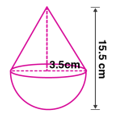Given radius of the cone, r = 3.5 cm
Radius of hemisphere, r = 3.5 cm
Total height of the toy = 15.5 cm
Height of the cone = 15.5 – 3.5 = 12 cm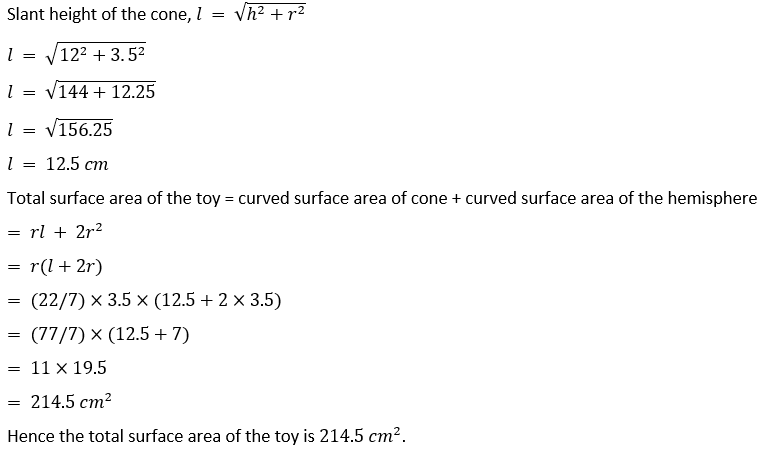10. A circus tent is in the shape of a cylinder surmounted by a cone. The diameter of the cylindrical portion is 24 m and its height is 11 m. If the vertex of the cone is 16 m above the ground, find the area of the canvas used to make the tent.
Solution: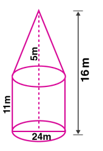Given diameter of the cylindrical part of tent, d = 24 m
Radius, r = d/2 = 24/2 = 12 m
Height of the cylindrical part, H = 11 m
Since vertex of cone is 16 m above the ground, height of cone, h = 16-11
h = 5 m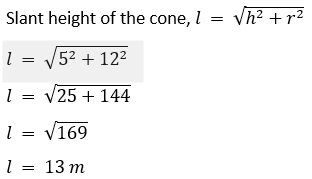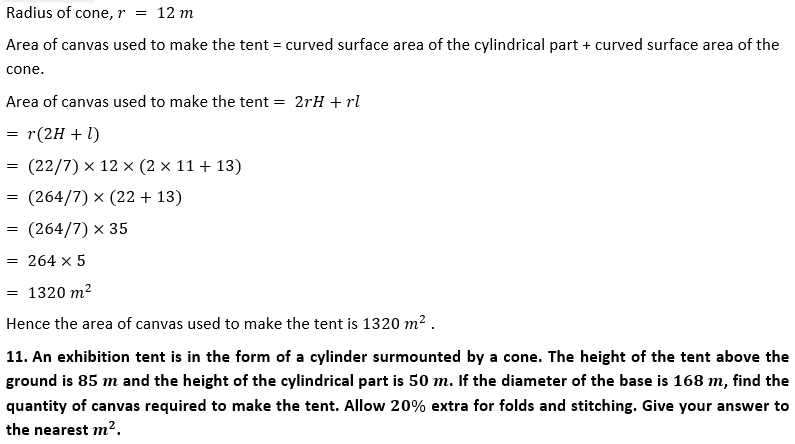Solution:
We have given that:Given height of the tent above the ground = 85 m
Height of the cylindrical part, H = 50 m
height of the cone, h = 85-50
h = 35 m
Diameter of the base, d = 168 m
Radius of the base of cylindrical part, r = d/2 = 168/2 = 84 m
Radius of cone, r = 84 m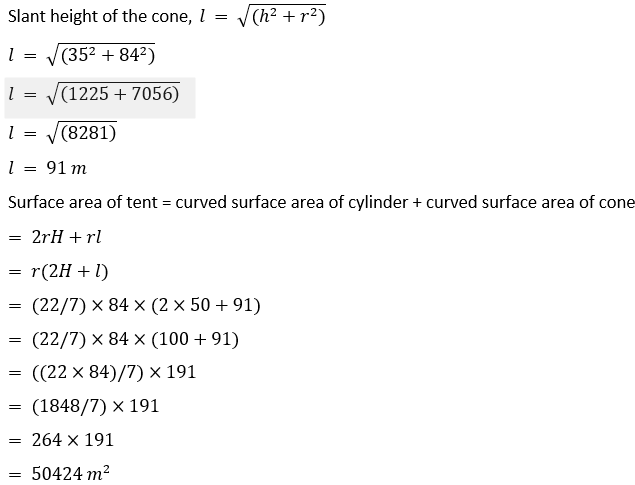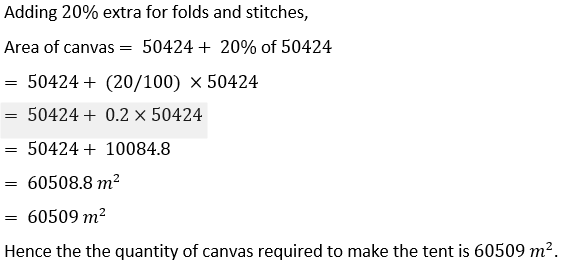12. From a solid cylinder of height 30 cm and radius 7 cm, a conical cavity of height 24 cm and of base radius 7 cm is drilled out. Find the volume and the total surface of the remaining solid.
Solution:
We have given that: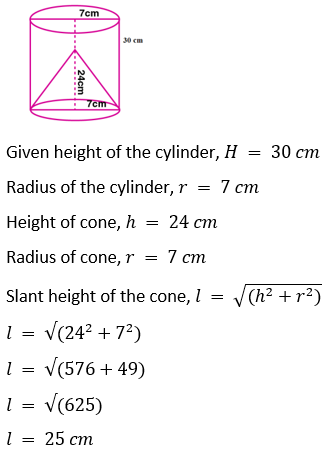Volume of the remaining solid = Volume of the cylinder – Volume of the cone
= r2 H – (1/3)r2h
= r2 (H- h/3)
= (22/7)×72×(30-24/3)
= (22×7)×(30-8)
= (154)×(22)
= 3388 cm3
Volume of the remaining solid is 3388 cm3.
Total surface area of the remaining solid = Curved surface area of cylinder + surface area of top of the cylinder+ curved surface area of the cone
Total surface area of the remaining solid = 2rH + r2  + rl
= r(2H + r + l)
=(22/7)×7(2×30 + 7+ 25)
= 22×(60+32)
= 22×92
= 2024 cm2
Hence the total surface area of the remaining solid is 2024 cm2.

13. The adjoining figure shows a wooden toy rocket which is in the shape of a circular cone mounted on a circular cylinder. The total height of the rocket is 26 cm, while the height of the conical part is 6 cm. The base of the conical portion has a diameter of 5 cm, while the base diameter of the cylindrical portion is 3 cm. If the conical portion is to be painted green and the cylindrical portion red, find the area of the rocket painted with each of these colours.
Also, find the volume of the wood in the rocket. Use π = 3.14 and give answers correct to 2 decimal places.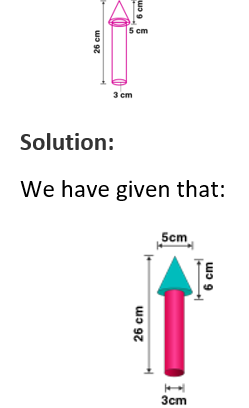(i) Given height of the rocket = 26 cm
Height of the cone, H = 6 cm
Height of the cylinder, h = 26-6 = 20 cm
Diameter of the cone = 5 cm
Radius of the cone, R = 5/2 = 2.5 cm
Diameter of the cylinder = 3 cm
Radius of the cylinder, r = 3/2 = 1.5 cm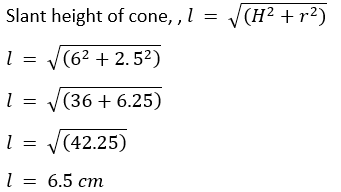Curved surface area of the cone = Rl
= 3.14×2.5×6.5
= 51.025 cm2
Base area of cone = R2
= 3.14×2.52
= 3.14×6.25
= 19.625 cm2
Curved surface area of the cylinder = 2rh
= 2×3.14×1.5×20
= 188.4 cm2
Base area of cylinder = r2
= 3.14×1.52
= 3.14×2.25
= 7.065 cm2
Total surface area of conical portion to be painted green = Curved surface area of the cone+ Base area of cone- Base area of cylinder
= 51.025 + 19.625 – 7.065
= 63.585 cm2
= 63.59 cm2
Hence the area of the rocket painted with green colour is 63.59 cm2 .
Total surface area of the cylindrical portion to be painted red = Curved surface area of the cylinder + Base area of cylinder
= 188.4+7.065
= 195.465 cm2
= 195.47 cm2
Hence the area of the rocket painted with red colour is 195.47 cm2 .
(ii) Volume of wood in the rocket = Volume of cone + Volume of cylinder
= (1/3)R2 H + r2 h
= ((R2 H/3) + r2 h))
= 3.14×((2.52×6/3) + 1.52×20)
= 3.14×((6.25×2) + 2.25×20)
= 3.14×(12.5+ 45)
= 3.14×57.5
= 180.55 cm3
Hence the volume of the wood in the rocket is 180.55 cm3

14. The adjoining figure shows a hemisphere of radius 5 cm surmounted by a right circular cone of base radius 5 cm. Find the volume of the solid if the height of the cone is 7 cm. Give your answer correct to two places of decimal.Solution:
We have given that:
Given radius of the hemisphere,r = 5 cm
Radius of cone, r = 5 cm
Height of the cone, h = 7 cm
Volume of the solid = Volume of the hemisphere + Volume of the cone
= (2/3)r3  + (1/3)r2 h
= (1/3)r2 (2r+h)
= (1/3)×(22/7)×52 (2×5+7)
= (22/21)×25(10+7)
= (22/21)×25×17
= 445.238 cm3
= 445.24  cm3
Hence the volume of the solid is 445.24  cm3 .

15. A buoy is made in the form of a hemisphere surmounted by a right cone whose circular base coincides with the plane surface of the hemisphere. The radius of the base of the cone is 3.5 metres and its volume is 2/3 of the hemisphere. Calculate the height of the cone and the surface area of the buoy correct to 2 places of decimal.
Solution:
We have given that: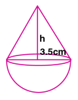Given radius of the cone,r = 3.5 cm
Radius of hemisphere, r = 3.5 cm = 7/2 cm
Volume of hemisphere = (2/3)r3
= (2/3)×(22/7)×(7/2)3
= (2/3)×(22/7)×(7/2)×(7/2)×(7/2)
= (22/3)×(7/2)×(7/2)
= 11×49/6
= 539/6 m3
Volume of cone = 2/3 of volume of hemisphere
= (2/3)× 539/6
= 539/9 m3
Volume of cone = (1/3)r2 h
(1/3)r2 h = 539/9
(1/3)×(22/7)×(7/2)2×h = 539/9
h = 539×3×2/9×11×7
h = 14/3
h = 4.667
h = 4.67 m
Hence the height of the cone is 4.67 m.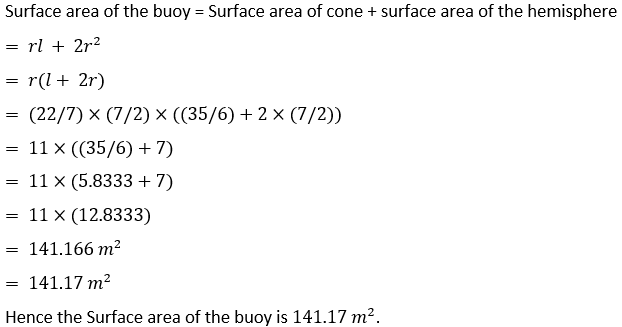16. A circular hall (big room) has a hemispherical roof. The greatest height is equal to the inner diameter, find the area of the floor, given that the capacity of the hall is 48510 m3.
Solution:
We have given that: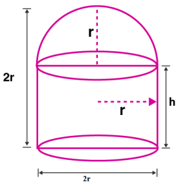Let the radius of the hemisphere be r.
Inner diameter = 2r
Given greatest height equal to inner diameter.
So total height of the hall = 2r
Height of the hemispherical part = r
Height of cylindrical area, h = 2r-r = r
Capacity of the hall = Volume of cylindrical area + volume of hemispherical area
= r^2 h + (2/3)r
= r+ (2/3)r [∵h = r]
= r (1+2/3)
= r (3+2)/3
= (5/3)r
Given capacity of hall = 48510 m3
(5/3)r= 48510
(5/3)×(22/7)r= 48510
r= 48510×3×7/(22×5)
r= 9261
Taking cube root on both sides,
r = 21
Area of the floor = r2
= (22/7)×212
= (22×21×3
= 1386 m2
Hence the Area of the floor is 1386 m2 .

17. A building is in the form of a cylinder surmounted by a hemisphere valted dome and contains 41 19/21 m3 of air. If the internal diameter of dome is equal to its total height above the floor, find the height of the building.
Solution:
We have given that:
Let the radius of the dome be r.
Internal diameter = 2r
Given internal diameter is equal to total height.
Total height of the building = 2r
Height of the hemispherical area = r
So height of cylindrical area, h = 2r-r = r
Volume of the building = Volume of cylindrical area + volume of hemispherical area
= r2 h + (2/3)r3
= r3+ (2/3)r3 [∵h = r]
= r3 (1+2/3)
= r3 (3+2)/3
= (5/3)r3
Given Volume of the building =
= 880/21
(5/3)r3= 880/21
(5/3)×(22/7)×r= 880/21
r3= 880×3×7/(5×22×21)
r3= 880/110
r3= 8
Taking cube root
r = 2 m
Height of the building = 2r = 2×2 = 4m
Hence the height of the building is 4m.

18. A rocket is in the form of a right circular cylinder closed at the lower end and surmounted by a cone with the same radius as that of the cylinder. The diameter and the height of the cylinder are 6 cm and 12 cm respectively. If the slant height of the conical portion is 5 cm, find the total surface area and the volume of the rocket. (Use π = 3.14).
Solution:
We have given that:Given diameter of the cylinder = 6 cm
Radius of the cylinder, r = 6/2 = 3 cm
Height of the cylinder, H = 12 cm
Slant height of the cone, l = 5 cm
Radius of the cone, r = 3 cm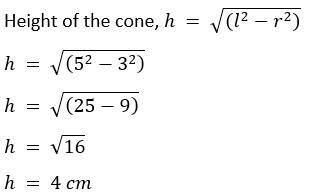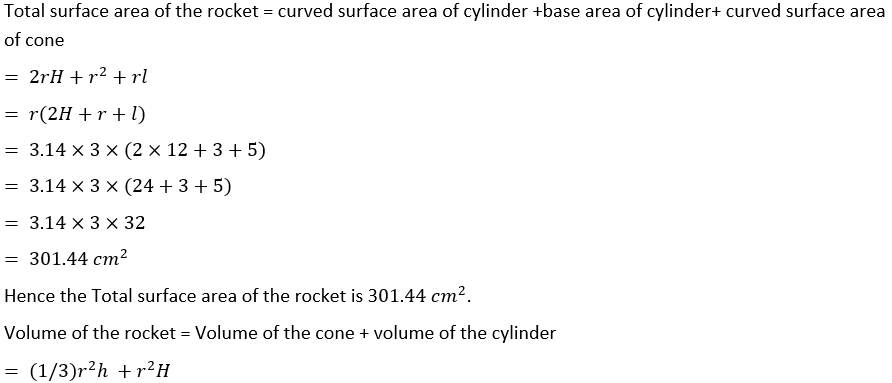= r2 ((h/3)+H)
= 3.14×32×((4/3)+12)
= 3.14×9×((4+36)/3)
= 3.14×9×(40/3)
= 3.14×3×40
= 376.8 cm3
Hence the volume of the rocket is 376.8  cm3.

19. The adjoining figure represents a solid consisting of a right circular cylinder with a hemisphere at one end and a cone at the other. Their common radius is 7 cm. The height of the cylinder and the cone are each of 4 cm. Find the volume of the solid.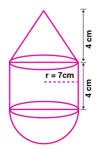Solution:
We have given that:
Given common radius, r = 7 cm
Height of the cone, h = 4 cm
Height of the cylinder, H = 4 cm
Volume of the solid = Volume of the cone + Volume of the cylinder + Volume of the hemisphere
= (1/3)r2 h +r2 H + (2/3) r3
= r2((h/3) + H + (2r/3))
= (22/7)×72×((4/3)+4+(2×7)/3)
= (22×7×((4+12+14)/3)
= 22×7×30/3
= 1540 cm3
Hence the volume of the solid is 1540 cm3.

20. A solid is in the form of a right circular cylinder with a hemisphere at one end and a cone at the other end. Their common diameter is 3.5 cm and the height of the cylindrical and conical portions are 10 cm and 6 cm respectively. Find the volume of the solid. (Take π = 3.14)
Solution:
We have given that:Given height of the cylinder, H = 10 cm
Height of the cone, h = 6 cm
Common diameter = 3.5 cm
Common radius, r = 3.5/2 = 1.75 cm
Volume of the solid = Volume of the cone + Volume of the cylinder + Volume of the hemisphere
= (1/3)r2 h +r2 H + (2/3) r3
= r2 ((h/3) + H + (2r/3))
= 3.14×1.752×((6/3)+10+(2×1.75)/3)
= 3.14×3.0625×(2+10+1.167)
= 3.14×3.0625×13.167
= 9.61625×13.167
= 126.617 cm3
= 126.62 cm3
Hence the volume of the solid is 126.62 cm3.

21. A toy is in the shape of a right circular cylinder with a hemisphere on one end and a cone on the other. The height and radius of the cylindrical part are 13 cm and 5 cm respectively. The radii of the hemispherical and conical parts are the same as that of the cylindrical part. Calculate the surface area of the toy if the height of the conical part is 12 cm.
Solution:
We have given that: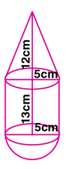Given height of the cylinder, H = 13 cm
Radius of the cylinder, r = 5 cm
Radius of the hemisphere, r = 5 cm
Height of the cone, h = 12 cm
Radius of the cone, r = 5 cmSurface area of the toy = curved surface area of cylinder +curved surface area of hemisphere+ curved surface area of cone
= 2rH+2r2+rl
= r(2H+2r+l)
= (22/7)×5(2×13+2×5+13)
= (110/7)×(26+10+13)
= (110/7)×49
= 110×7
= 770 cm2
Hence the surface area of the toy is 7770 cm2.

22.The adjoining figure shows a model of a solid consisting of a cylinder surmounted by a hemisphere at one end. If the model is drawn to a scale of 1∶ 200, find
(i) the total surface area of the solid in π m².
(ii) the volume of the solid in π litres.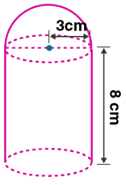Solution:
We have given that:
Given height of the cylinder, h = 8 cm
Radius of the cylinder, r = 3 cm
Radius of hemisphere , r = 3 cm
Scale = 1:200
Hence actual radius, r = 200×3 = 600
Actual height, h = 200×8 = 1600
(i)Total surface area of the solid = Base area of the cylinder + Curved surface area of the cylinder + curved surface area of the hemisphere
= r2+2rh + 2r2
= r(r+2h+2r)
= ×600(600+2×1600+2×600)
= 600 ×(600+3200+1200)
= 600 ×(5000)
= 3000000 cm2
= 300 m2
Hence the total surface area of the solid is 300 m2.
(ii)Volume of the solid = Volume of the cylinder + Volume of the hemisphere
= r2 h + (2/3) r3
= r2 (h+ (2/3)r)
= × 6002 (1600+ (2/3)×600)
= 360000 (1600+400)
= 360000 ×2000
= 720000000 cm3
= 720 m3
= 720000 litres [1 m3  = 1000 litres]
Hence the volume of the solid is 720000 litres.

Exercise 17.5

1. The diameter of a metallic sphere is 6 cm. The sphere is melted and drawn into a wire of uniform cross-section. If the length of the wire is 36 m, find its radius.
Solution:
We have given that:
Given diameter of the metallic sphere = 6 cm
Radius of the sphere, r = 6/2 = 3 cm
Volume of the sphere, V = (4/3)r3
= (4/3)××33
= 4××9
= 36 cm3
Length of the wire, h = 36 m = 3600 cm
Since the sphere is melted and drawn into a wire, volume remains the same.
Volume of the wire, r2 h = 36
r2×3600 = 36
r= 1/100
r = 1/10 = 0.1 cm = 1 mm
Hence the radius of the wire is 1 mm.

2. The radius of a sphere is 9 cm. It is melted and drawn into a wire of diameter 2 mm. Find the length of the wire in metres.
Solution:
We have given that:
Radius of the sphere, r = 9 cm
Volume of the sphere, V = (4/3)r3
= (4/3)××93
= 12××81
= 972 cm3
Diameter of the wire = 2 mm
So radius of the wire = 2/2 = 1 mm = 0.1 cm
Since the sphere is melted and drawn into a wire, volume remains the same.
Volume of the wire, r2 h = 972
×0.12×h =972
h = 972/0.12
h = 972/0.01
h = 97200 cm
h = 972 m
Hence the length of the wire is 972 m.

3. A solid metallic hemisphere of radius 8 cm is melted and recasted into right circular cone of base radius 6 cm. Determine the height of the cone.
Solution:
We have given that:
Given radius of the hemisphere, r = 8 cm
Volume of the hemisphere, V = (2/3)r3
= (2/3)×83
= (1024/3) cm3
Radius of cone, R = 6 cm
Since hemisphere is melted and recasted into a cone, the volume remains the same.
Volume of the cone, (1/3)R2 h = (1024/3)
(1/3)×62  ×h = (1024/3)
36h = 1024
h = 1024/36
= 28.44 cm
Hence the height of the cone is 28.44 cm.

4. A rectangular water tank of base 11 m × 6 m contains water upto a height of 5 m. if the water in the tank is transferred to a cylindrical tank of radius 3.5 m, find the height of the water level in the tank.
Solution:
We have given that:
Given dimensions of the rectangular water tank = 11 m × 6 m
Height of water in tank = 5 m
Volume of water in tank = 11×6×5 = 330 m3
Radius of the cylindrical tank, r = 3.5 m
Volume of cylindrical tank = r2 h
r2 h = 330
(22/7)×3.52×h = 330
(22/7)×12.25×h = 330
h = 330×7/22×12.25
= 8.57 m
Hence the height of the water level in the tank is 8.57m.

5. The rain water from a roof of dimensions 22 m × 20 m drains into a cylindrical vessel having diameter of base 2 m and height; 3.5 m. If the rain water collected from the roof just fill the cylindrical vessel, then find the rainfall in cm.
Solution:
We have given that:
Given dimensions of the cylindrical vessel = 22 m × 20 m
Let the rainfall be × cm.
Volume of water = (22×20×X)m3
Diameter of the cylindrical base = 2 m
So radius of the cylindrical base = 2/2 = 1 m
Height of the cylindrical base, h = 3.5 m
Volume of cylindrical vessel = r2h
= (22/7)×12×3.5
= 11 m3
Since the rain water collected from the roof just fill the cylindrical vessel, the volumes are equal.
22×20×X = 11
X= 11/22×20
= 1/40 m
= (1/40)×100 cm
= 2.5 cm
Hence the rainfall is 2.5 cm.

6. The volume of a cone is the same as that of the cylinder whose height is 9 cm and diameter 40 cm. Find the radius of the base of the cone if its height is 108 cm.
Solution:
We have given that:
Given height of the cylinder, h = 9 cm
Diameter of the cylinder = 40 cm
Radius of the cylinder, r = 40/2= 20 cm
Volume of the cylinder = r2h
= × 202×9
= ×400×9
= 3600 cm3
Height of the cone, H = 108 cm
Volume of cone = (1/3)r2h
= (1/3)r2×108
= 36r2
Since volume of cone is equal to the volume of the cylinder, we get
36r2  = 3600
r2  = 3600/36
r2  = 100
Taking square root on both sides,
r = 10 cm
Hence the radius of the cone is 10 cm.

7. Eight metallic spheres, each of radius 2 cm, are melted and cast into a single sphere. Calculate the radius of the new (single) sphere.
Solution:
We have given that:
Given radius of each sphere,r = 2 cm
Volume of a sphere = (4/3)r3
= (4/3)×23
= (4/3)×8
= (32/3) cm3
Volume of 8 spheres = 8×(32/3)
= (256/3) cm3
Let R be radius of new sphere.
Volume of the new sphere = (4/3)R3
Since 8 spheres are melted and casted into a single sphere, volume remains same.
(4/3)R3= (256/3)
4R3  = 256
R3= 256/4 = 64
Taking cube root
R = 4 cm
Hence the radius of the new sphere is 4 cm.

8. A metallic disc, in the shape of a right circular cylinder, is of height 2.5 mm and base radius 12 cm. Metallic disc is melted and made into a sphere. Calculate the radius of the sphere.
Solution:
We have given that:
Given height of the cylinder, h = 2.5 mm = 0.25 cm
Radius of the cylinder, r = 12 cm
Volume of the cylinder = r2h
= × 122×0.25
= ×144×0.25
= 36 cm3
Let R be the radius of the sphere.
Volume of sphere = (4/3)R3
Since metallic disc is melted and made into a sphere, their volumes remains same.
(4/3)R= 36
R3= 36×3/4
R3= 27
Taking cube root
R = 3 cm
Hence the radius of the sphere is 3 cm.

9. Two spheres of the same metal weigh 1 kg and 7 kg. The radius of the smaller sphere is 3 cm. The two spheres are melted to form a single big sphere. Find the diameter of the big sphere.
Solution:
We have given that:
For same material, density will be same.
Density = mass/Volume
Mass of the smaller sphere, m = 1 kg
Mass of the bigger sphere, m2  = 7 kg
The spheres are melted to form a new sphere.
So the mass of new sphere, m3  = 1+7 = 8 kg
Density of smaller sphere = density of new sphere
Let V1 be volume of smaller sphere and V3 be volume of bigger sphere.
m1/V1  = m3/V3
1/V1= 8/V3
V1/ V3= 1/8 …(i)
Given radius of the smaller sphere, r = 3 cm
V= (4/3)r3
V1= (4/3) ××33
V1= 36
Let R be radius of new sphere.
V3 = (4/3)R^3
V1/ V_3= 36÷(4/3)R3
V1/ V_3= 27/R3 …(ii)
From (i) and (ii)
1/8 = 27/R3
R3= 27×8 = 216
Taking cube root on both sides,
R = 6 cm
So diameter of the new sphere = 2R = 2×6 = 12 cm
Hence diameter of the new sphere is 12 cm.

10. A hollow copper pipe of inner diameter 6 cm and outer diameter 10 cm is melted and changed into a solid circular cylinder of the same height as that of the pipe. Find the diameter of the solid cylinder.
Solution:
We have given that:
Given inner diameter of the pipe = 6 cm
So inner radius, r = 6/2 = 3 cm
Outer diameter = 10 cm
Outer radius, R = 10/2 = 5 cm
Let h be the height of the pipe.
Volume of pipe = (R2-r2)h
= ×(52-32)×h
= h(25-9)
= 16h cm3
Let r be the radius of solid cylinder.
Volume of solid cylinder = r2h
Since pipe is melted and changed into a cylinder, their volumes remains same.
r2h = 16h
r2  = 16
r = 4 cm
Diameter = 2r = 2×4 = 8 cm
Hence the diameter of the cylinder is 8 cm.

11. A solid sphere of radius 6 cm is melted into a hollow cylinder of uniform thickness. If the external radius of the base of the cylinder is 4 cm and height is 72 cm, find the uniform thickness of the cylinder.
Solution:
We have given that:
Given radius of the sphere, r = 6 cm
Volume of the sphere = (4/3)r3
= (4/3)×63
= 288 cm3
Let r be the internal radius of the hollow cylinder.
External radius of the hollow cylinder, R = 4 cm
Height of hollow cylinder, h = 72 cm
Volume of hollow cylinder = (R2-r2)h
Since sphere is melted and changed into a hollow cylinder, their volumes remain same.
(R2-r2)h = 288
(42-r2)×72 = 288
(42-r2) = 288/72
(42-r2) = 4
16-r2= 4
r2= 16-4
r2= 12
r = 2√3  cm
So thickness = R-r = 4-2√3
= 4- 3.464
= 0.536 cm
= 0.54 cm (approx)
Hence the thickness of the cylinder is 0.54 cm.

12. A hollow metallic cylindrical tube has an internal radius of 3 cm and height 21 cm. The thickness of the metal of the tube is ½ cm. The tube is melted and cast into a right circular cone of height 7 cm. Find the radius of the cone correct to one decimal place.
Solution:
We have given that:
Given internal radius of the tube, r = 3 cm
Thickness of the tube = ½ cm = 0.5 cm
External radius of tube = 3+0.5 = 3.5 cm
Height of the tube, h = 21 cm
Volume of the tube = (R2-r2)h
= (3.52-32)×21
= (12.25-9)×21
= (3.25)×21
= 68.25 cm3
Height of the cone, h = 7 cm
Let r be radius of cone.
Volume of cone = (1/3)r2 h
= (1/3)r2×7
= (7/3)r2
Since tube is melted and changed into a cone, their volumes remain same.
(7/3)r2= 68.25
r2= 68.25×3/7 = 29.25
Taking square root on both sides
r = 5.4 cm
Hence the radius of the cone is 5.4 cm.

13. A hollow sphere of internal and external diameters 4 cm and 8 cm respectively, is melted into a cone of base diameter 8 cm. Find the height of the cone. (2002)
Solution:
We have given that:
Given internal diameter of hollow sphere = 4 cm
Internal radius, r = 4/2 = 2 cm
External diameter = 8 cm
External radius, R = 8/2 = 4 cm
Volume of the hollow sphere, V = (4/3)(R3-r3)
V = (4/3)×(43-23)
V = (4/3)×(64-8)
V = (4/3)×56
Base diameter of the cone = 8 cm
Radius, r = 8/2 = 4 cm
Volume of cone = (1/3)r2h
= (1/3)×42  ×h
= (16/3)×h
Since sphere is melted and changed into a cone, their volumes remain same.
(4/3)×56 = (16/3)×h
h = 4×56/16
h = 14 cm
Hence the height of the cone is 14 cm.

14. A well with inner diameter 6 m is dug 22 m deep. Soil taken out of it has been spread evenly all round it to a width of 5 m to form an embankment. Find the height of the embankment.
Solution:
We have given that: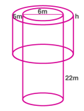Given inner diameter of the well = 6 m
Radius of the well, r = 6/2 = 3 m
Depth of the well, H = 22 m
Volume of the soil dug out of well = r2H
= ×32×22
= 198 m3
Width of the embankment = 5 m
Inner radius of embankment, r = 3 m
Outer radius of embankment, R = 3+5 = 8 m
Let h be height of the soil embankment.
Volume of the soil embankment = (R2– r2)h
= (82– 32)h
= (64-9)h
= 55h
Volume of the soil dug out = volume of the soil embankment
198 = 55h
h = 198/55
h = 3.6 m
Hence the height of the soil embankment is 3.6 m.

15. A cylindrical can of internal diameter 21 cm contains water. A solid sphere whose diameter is 10.5 cm is lowered into the cylindrical can. The sphere is completely immersed in water. Calculate the rise in water level, assuming that no water overflows.
Solution:
We have given that:Given internal diameter of cylindrical can = 21 cm
Radius of the cylindrical can, R = 21/2 cm
Diameter of sphere = 10.5 cm
Radius of the sphere, r = 10.5/2 = 21/4 cm
Let the rise in water level be h.
Rise in volume of water = Volume of sphere immersed
R2 h = (4/3)r3
×(21/2)2 h = (4/3)××(21/4)3
(21/2) ×(21/2)×h = (4/3)× (21/4)×(21/4)× (21/4)
h = 21/12
h = 7/4
h = 1.75 cm
Hence the rise in water level is 1.75 cm.

16. There is water to a height of 14 cm in a cylindrical glass jar of radius 8 cm. Inside the water there is a sphere of diameter 12 cm completely immersed. By what height will the water go down when the sphere is removed?
Solution:
We have given that:Given radius of the glass jar, R = 8 cm
Diameter of the sphere = 12 cm
Radius of the sphere, r = 12/2 = 6 cm
When the sphere is removed from the jar, volume of water decreases.
Let h be the height by which water level will decrease.
Volume of water decreased = Volume of the sphere
R2 h = (4/3)r3
82 h = (4/3)6^3
h = (4/3)×6×6×6/(8×8)
= 18/4 = 9/2 = 4.5 cm
Hence the height by which water level decreased is 4.5 cm.

17. A vessel in the form of an inverted cone is filled with water to the brim. Its height is 20 cm and diameter is 16.8 cm. Two equal solid cones are dropped in it so that they are fully submerged. As a result, one-third of the water in the original cone overflows. What is the volume of each of the solid cone submerged?
Solution:
We have given that: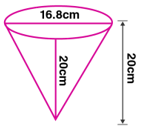Given height of the cone, h = 20 cm
Diameter of the cone = 16.8 cm
Radius of the cone, r = 16.8/2 = 8.4 cm
Volume of water in the vessel = (1/3)r2 h
= (1/3)×8.42  ×20
= (1/3)×(22/7)×8.4 ×8.4 ×20
= 1478.4 cm3
One third of volume of water in the vessel = (1/3)× 1478.4
= 492.8 cm3
One third of volume of water in the vessel = Volume of water over flown
Volume of water over flown = volume of two equal solid cones dropped into the vessel.
volume of two equal solid cones dropped into the vessel = 492.8 cm3
Volume of one solid cone dropped into the vessel = 492.8/2 = 246.4 cm3
Hence the volume of each of the solid cone submerged is 246.4 cm3.

18. A solid metallic circular cylinder of radius 14 cm and height 12 cm is melted and recast into small cubes of edge 2 cm. How many such cubes can be made from the solid cylinder?
Solution:
Radius of the solid circular cylinder, r = 14 cm
Height, h = 12 cm
Volume of the cylinder = r2 h
= ×142×12
= ×196×12
= 2352
= 2352×22/7
= 7392 cm3
Edge of the cube, a = 2 cm
Volume of cube = a3
= 23  = 8 cm3
Number of cubes made from solid cylinder = 7392/8 = 924.
Hence the number of cubes made from solid cylinder is 924.

19. How many shots each having diameter 3 cm can be made from a cuboidal lead solid of dimensions 9 cm × 11 cm × 12 cm?
Solution:
We have given that:
Given dimensions of the cuboidal solid = 9 cm× 11 cm× 12 cm
Volume of the cuboidal solid = 9×11×12 = 1188  cm3
Diameter of shot = 3 cm
So radius of shot, r = 3/2 = 1.5 cm
Volume of shot = (4/3)r3
= (4/3)×1.53
= (4/3)×(22/7)×1.53
= 297/21 cm3
= 1188×21/297
= 84
Hence the number of shots made from cuboidal lead of solid is 84.

20. How many spherical lead shots of diameter 4 cm can be made out of a solid cube of lead whose edge measures 44 cm?
Solution:
We have given that:
Edge of the cube, a = 44 cm
Volume of cube = a3
= 44
= 85184 cm
Diameter of shot = 4 cm
So radius of shot, r = 4/2 = 2 cm
Volume of a shot = (4/3)r3
= (4/3)×(22/7)×23
= 704/21 cm3
= 85184×21/704
= 2541
Hence the number of shots made from cube is 2541.

21. Find the number of metallic circular discs with 1.5 cm base diameter and height 0.2 cm to be melted to form a circular cylinder of height 10 cm and diameter 4.5 cm.
Solution:
We have given that:
Given height of the circular cylinder, h = 10 cm
Diameter of circular cylinder = 4.5 cm
So radius, r = 4.5/2 = 2.25 cm
Volume of circular cylinder = r2h
= ×2.252×10 = 50.625 cm3
Base diameter of circular disc = 1.5 cm
So radius, r = 1.5/2 = 0.75 cm
Height of the circular disc, h = 0.2 cm
Volume of circular disc = r2h
= ×0.752  ×0.2
= 0.1125 cm3
Number of circular discs made from cylinder = 50.625/0.1125 = 450
Hence the number of circular discs made from cylinder is 450.

22. A solid metal cylinder of radius 14 cm and height 21 cm is melted down and recast into spheres of radius 3.5 cm. Calculate the number of spheres that can be made.
Solution:
We have given that:
Given radius of the metal cylinder,r = 14 cm
Height of the metal cylinder, h = 21 cm
Radius of the sphere, R = 3.5 cm
Volume of the metal cylinder = r2h
= (22/7)×142×21
= 22×2×14×21
= 12936 cm3
Volume of sphere = (4/3)R3
= (4/3)×(22/7)×3.53
= 11×49/3
= 539/3 cm3
Number of spheres that can be made = Volume of the metal cylinder/ Volume of sphere
= 12936 ÷539/3
= 12936 ×3/539
= 72
Hence the number of spheres that can be made is 72.

23. A metallic sphere of radius 10.5 cm is melted and then recast into small cones, each of radius 3.5 cm and height 3 cm. Find the number of cones thus obtained.
Solution:
We have given that:
Given radius of the metallic sphere, R = 10.5 cm
Volume of the sphere = (4/3)R3
= (4/3)×10.53
= 1543.5 cm3
Radius of cone, r = 3.5 cm
Height of the cone, h = 3 cm
Volume of the cone = (1/3)r2h
= (1/3)×3.52×3
= 12.25 cm3
Number of cones made from sphere = Volume of sphere / volume of cone
= 1543.5 /12.25
= 126
Hence the number of cones that can be made is 126.

24. A certain number of metallic cones each of radius 2 cm and height 3 cm are melted and recast in a solid sphere of radius 6 cm. Find the number of cones.
Solution:
We have given that:
Given radius of metallic cones,r = 2 cm
Height of cone, h = 3 cm
Volume of cone = (1/3)r2h
= (1/3)×22×3
= 4 cm3
Radius of the solid sphere, R = 6 cm
Volume of the solid sphere = (4/3)R3
= (4/3)63
= 4××2×6×6
= 288 cm3
Number of cones made from sphere = Volume of solid sphere / volume of the cone
= 288/4
= 72
Hence the number of cones that can be made is 72.

25. A vessel is in the form of an inverted cone. Its height is 11 cm and the radius of its top, which is open, is 2.5 cm. It is filled with water upto the rim. When some lead shots, each of which is a sphere of radius 0.25 cm, are dropped into the vessel, 2/5 of the water flows out. Find the number of lead shots dropped into the vessel.
Solution:
We have given that:
Given height of the cone, h = 11 cm
Radius of the cone, r = 2.5 cm
Volume of the cone = (1/3)r2h
= (1/3)×2.52×11
= (11/3)×6.25 cm3
When lead shots are dropped into vessel, (2/5) of water flows out.
Volume of water flown out = (2/5)×(11/3)×6.25
= (22/15)×6.25
= (137.5/15) cm3
Radius of sphere, R = 0.25 cm = ¼ cm
Volume of sphere = (4/3)R3
= (4/3)×(1/4)3
= /48 cm3
Number of lead shots dropped = Volume of water flown out/ Volume of sphere
= (137.5/15) ÷48
= (137.5/15) ×48
= 137.5×48/15
= 440
Hence the number of lead shots dropped is 440.

26. The surface area of a solid metallic sphere is 616 cm². It is melted and recast into smaller spheres of diameter 3.5 cm. How many such spheres can be obtained?
Solution:
We have given that:
Given surface area of the sphere = 616 cm2
4R2  = 616
4×(22/7)R2= 616
R2= 616×7/4×22
R2= 49
R = 7
Volume of the solid metallic sphere = (4/3)R3
= (4/3)×73
= (1372/3) cm3
Diameter of smaller sphere = 3.5 cm
So radius, r = 3.5/2 = 7/4 cm
Volume of the smaller sphere = (4/3)r3
= (4/3)×(7/4)3
= (343/48) cm3
Number of spheres made = Volume of the solid metallic sphere/ Volume of the smaller sphere
= (1372/3) ÷(343/48)
=1372×48/(3×343)
= 64
Hence the number of spheres made is 64.

27. The surface area of a solid metallic sphere is 1256 cm². It is melted and recast into solid right circular cones of radius 2.5 cm and height 8 cm. Calculate (i) the radius of the solid sphere. (ii) the number of cones recast. (Use π = 3.14).
Solution:
We have given that:
(i)Given surface area of the solid metallic sphere = 1256 cm2
4R2  = 1256
4×3.14×R2= 1256
R= 1256/4×3.14
R= 100
R = 10
Hence the radius of solid sphere is 10 cm.
(ii)Volume of the solid sphere = (4/3)R3
= (4/3)×103
= (4000/3) cm3
= 12560/3  cm3
Radius of the cone,r = 2.5 cm
Height of the cone, h = 8 cm
Volume of the cone = (1/3)r2 h
= (1/3)×3.14×2.5^2×8
= 157/3 cm^3
Number of cones made = Volume of the solid sphere/ Volume of the cone
= (12560/3)÷( 157/3)
= (12560/3)×( 3/157)
= 12560/157
= 80
Hence the number of cones made is 80.

28. Water is flowing at the rate of 15 km/h through a pipe of diameter 14 cm into a cuboid pond which is 50 m long and 44 m wide. In what time will the level of water in the pond rise by 21 cm?
Solution:
We have given that:
Given speed of water flow = 15 km/h
Diameter of pipe = 14 cm
So radius of pipe, r = 14/2 = 7 cm = 0.07 m
Dimensions of cuboidal pond = 50 m × 44 m
Height of water in pond = 21 cm = 0.21 m
So volume of water in pond = 50×44 ×0.21
= 462 m
Volume of water in pipe = r2 h
= ×0.072×h
= 0.0049h
Volume of water in pond = Volume of water in pipe
462 = 0.0049h
h = 462/0.0049
= 462×7/0.0049×22
= 30000 m
= 30 km [1 km = 1000 m]
Speed = distance/ time
Time = Distance/speed = 30/15 = 2 hr
Hence the time taken is 2 hours.

29. A cylindrical can whose base is horizontal and of radius 3.5 cm contains sufficient water so that when a sphere is placed in the can, the water just covers the sphere. Given that the sphere just fits into the can, calculate : (i) the total surface area of the can in contact with water when the sphere is in it. (ii) the depth of the water in the can before the sphere was put into the can. Given your answer as proper fractions.
Solution:
We have given that: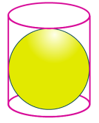(i)Given radius of the cylinder, r = 3.5 cm
Diameter of the sphere = height of the cylinder
= 3.5×2
= 7 cm
So radius of sphere, r = 7/2 = 3.5 cm
Height of cylinder, h = 7 cm
Total surface area of can in contact with water = curved surface area of cylinder+base area of cylinder.
= 2rh+r2
= r(2h+r)
= 22/7)×3.5×(2×7+3.5)
= (22/7)×3.5×(14+3.5)
= 11×17.5
= 192.5 cm2
Hence the surface area of can in contact with water is 192.5 cm2.
(ii) Let the depth of the water before the sphere was put be d.
Volume of cylindrical can = volume of sphere+ volume of water
r2  h = (4/3)r3+r2  d
r2  h = r2 {(4/3)r +d)}
h = (4/3)r +d
d = h – (4/3)r
d = 7 – (4/3)×3.5
d = (21-14)/3
d = 7/3
Hence the depth of the water before the sphere was put into the can is 7/3 cm.